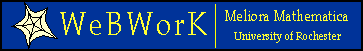## Department of Mathematics Computer and Tutoring Labs

There is a student computer lab in 155c MC (located between LCB and JWB) with computer support staff, internet access and printing facilities.

## The Preview Button

The preview button is your friend! Click on it and WeBWorK will tell you what it thinks you entered. This may be different from what you think, and seeing the difference may resolve your question!

## WeBWork Arithmetic

WeBWorK understands the following symbols:
 + Sum, for example you can write a+b. - difference, for example a-b. * product, for example a*b. / division, for example a/b. ^ or ** exponentiation, for example 3**4 (which equals 81), or 3**(1/3) for the cube root of 3.
Exponentiation comes first, multiplication and division next, and addition and subtraction last. You can overrule this sequence with parentheses. Expressions in parentheses are evaluated first. Redundant parentheses are legal and possible, use them to make your meaning clear.

```a + b
-----
c - d
```
enter it as (a+b)/(c-d).

Usually you can enter numerical answers as arithmetic expressions, for example, 1/7 instead of 0.142857.

Note that lower and upper case variables are different and usually cannot be interchanged with each other.

## WeBWorK Built-In Functions

The following is a list of functions and constants that are recognized by WeBWorK

 Function Description Constants: e Natural exponential (2.71828...) pi Pi, the ratio of the circumference to diameter of a circle (3.14159...) Miscellaneous functions: abs() absolute value exp() Natural exponential, e^() log() natural logarithm, same as ln ln() Natural logarithm, same as log sqrt() Square Root Function Trig functions: sin() sine of an angle (in radians) asin() inverse sine function cos() cosine of an angle (in radians) acos() inverse cosine function tan() tangent of an angle (in radians) atan() inverse tangent function sec() secant of an angle, 1/cos() cot() cotangent of an angle, 1/tan()